2021-01-14 17:19发布

# 正文

```<script>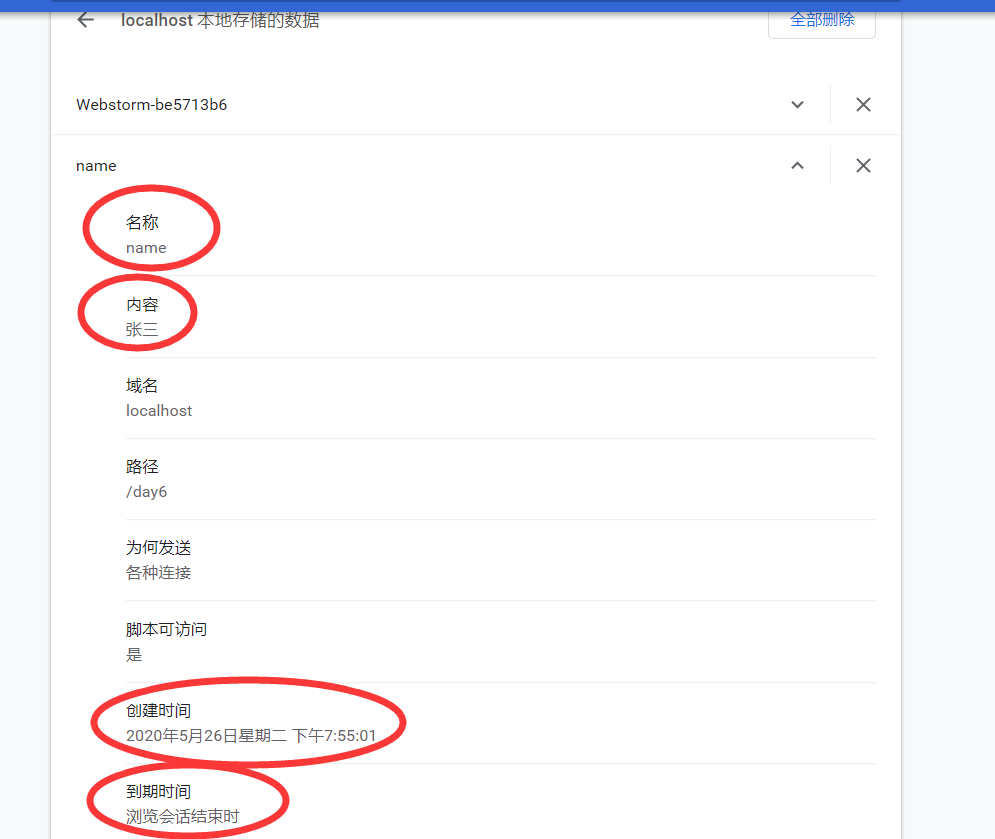```<script>
let data = new Date().getTime()   //先将此时的时间转化为毫秒
let new_data = new Date(data + 7*24*60*60*1000)  //将过期时间设置为7天后
// toUTCString() 是将时间根据世界时转换为字符串
document.cookie = 'name=张三;' + 'expires=' + new_data.toUTCString()</script>123456```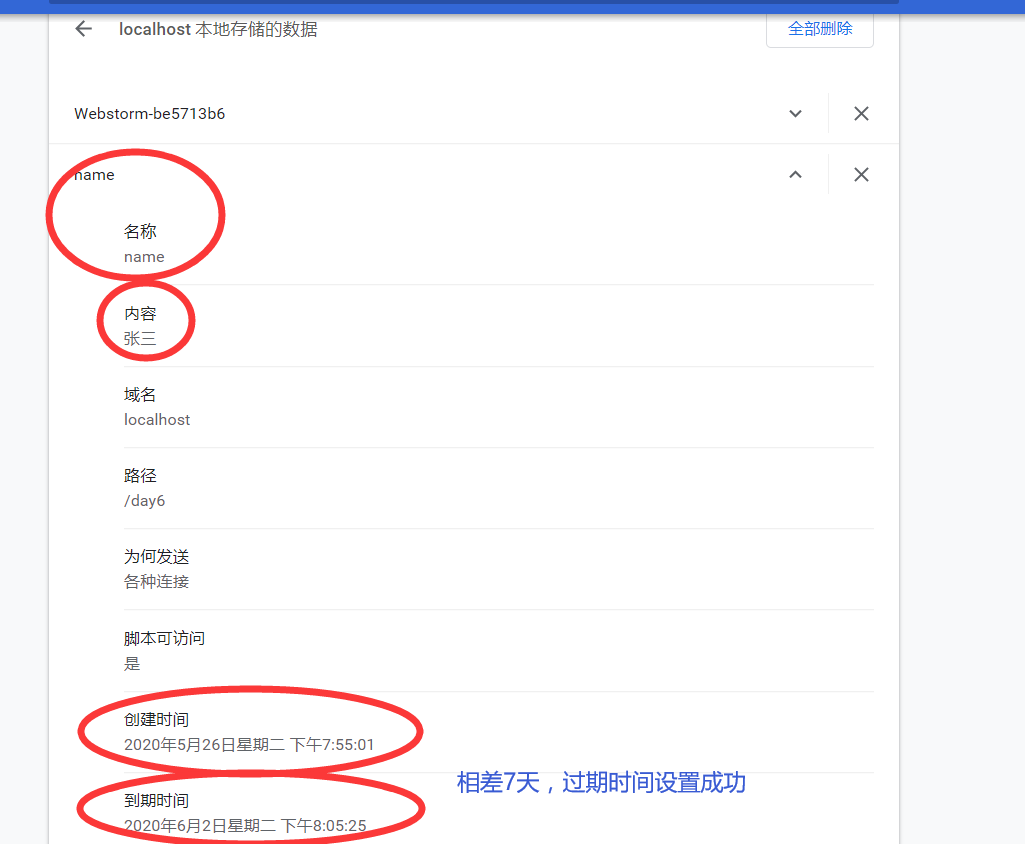```<script>
let data = new Date().getTime()   //先将此时的时间转化为毫秒
let new_data = new Date(data + 7*24*60*60*1000)  //将过期时间设置为7天后
document.cookie = 'name=张三;' + 'expires=' + new_data.toUTCString()
document.cookie = 'age=19;' + 'expires=' + new_data.toUTCString()</script>12345678```

```<script>

</script>12345```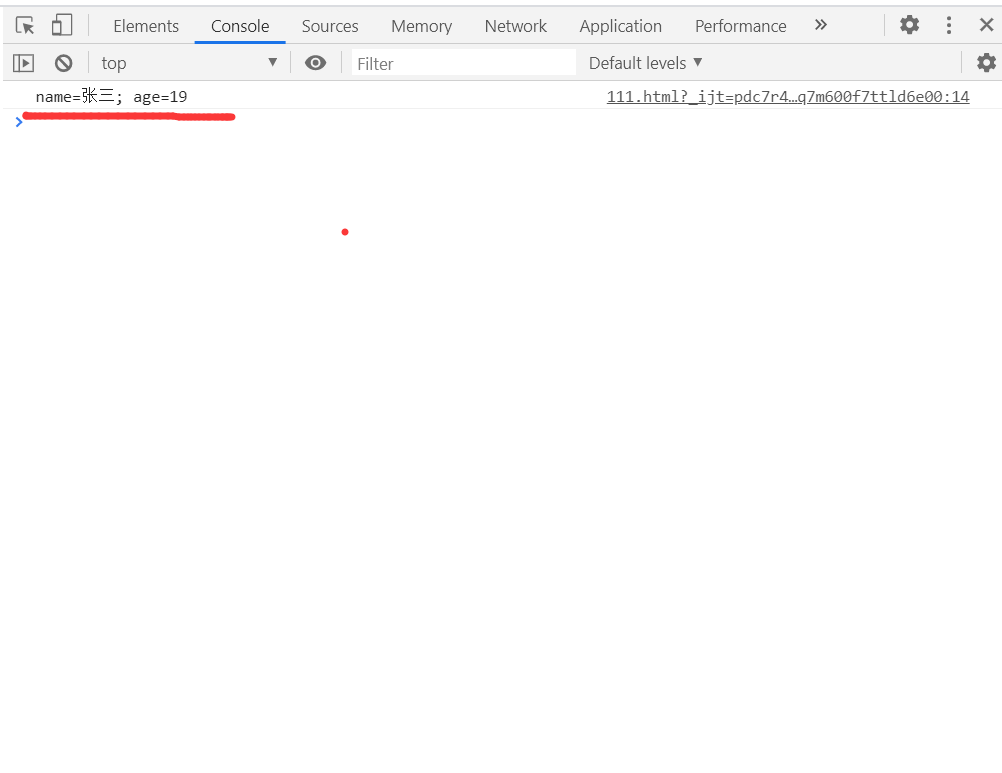```<script>
// name=张三 ; age=19

let re = new RegExp("\s?" + "name" + "=([^;]+)(;|\$)")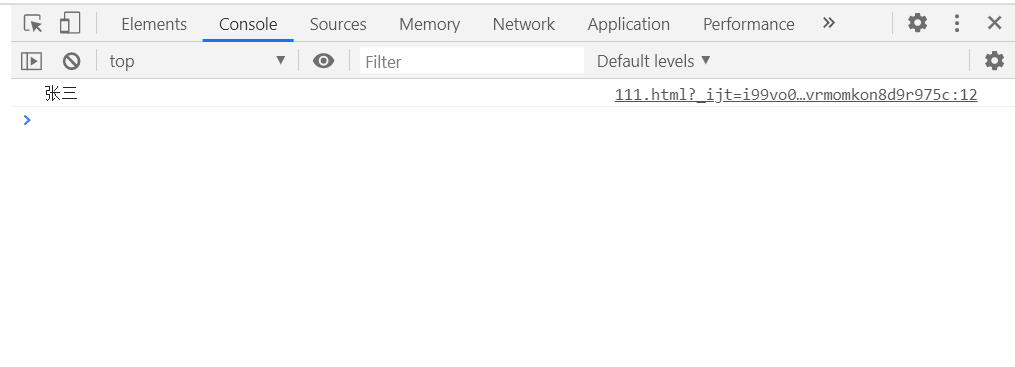```<script>
let data = new Date().getTime()   //先将此时的时间转化为毫秒
let old_data = new Date(data - 24*60*60*1000) //将过期时间设为24小时前
document.cookie = 'name=张三;' + 'expires=' + old_data.toUTCString()</script>12345```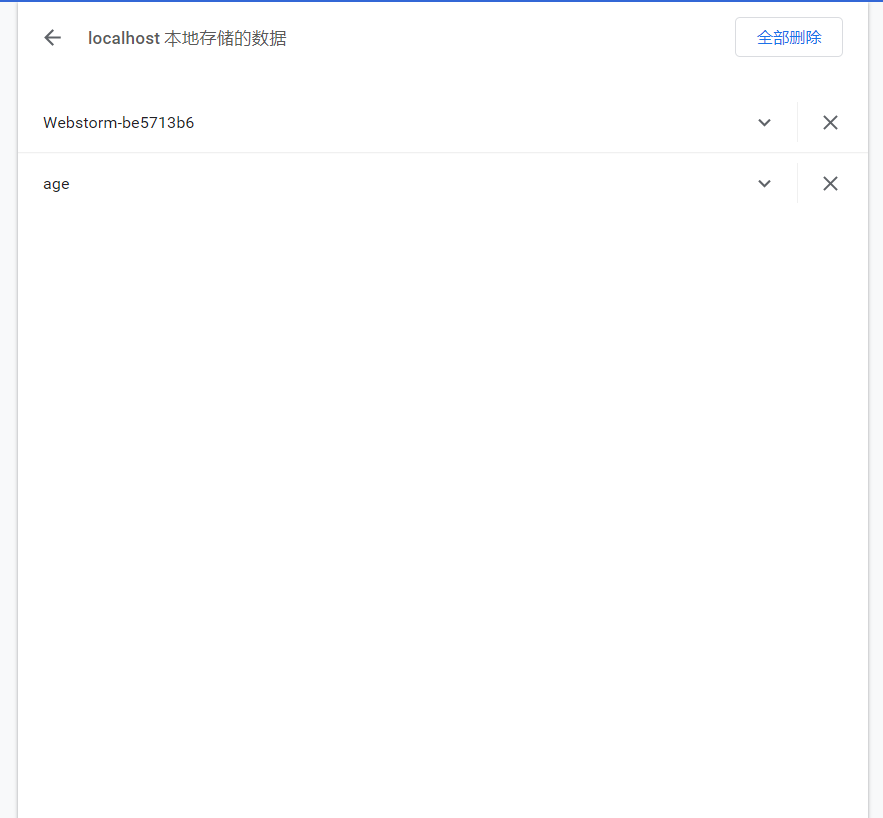```<script>

function setCookies(obj, limitTime) {
let data = new Date( new Date().getTime() + limitTime*24*60*60*1000 ).toUTCString()

for(let i in obj) {
document.cookie = i + '=' + obj[i] + ';expires=' + data	     }

}
</script>12345678910111213```

```// 第一个参数为对象，用键值对的形式传入我们想设置的cookie名和值// 第二个参数为过期时间，单位为天setCookies({
name: '张三',
age: 19,
like: '羽毛球'
}, 7)1234567```

```//查看cookie
let re = new RegExp("\s?" + cookieName + "=([^;]+)(;|\$)")
}12345```

`// 该函数只有一个参数，即我们需要查询的cookie名称，然后返回一个值let cookieValue = searchCookie(name)console.log(cookieValue)// 张三12345`

```//删除cookie
`//该函数只有一个参数，传入一个数组，每个元素为我们想要删除的cookie名removeCookies(['name', 'age'])12`